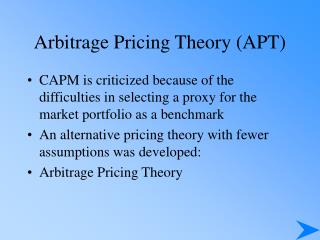DownloadDownload PresentationArbitrage Pricing Theory (APT)

# Arbitrage Pricing Theory (APT)

Télécharger la présentation## Arbitrage Pricing Theory (APT)

- - - - - - - - - - - - - - - - - - - - - - - - - - - E N D - - - - - - - - - - - - - - - - - - - - - - - - - - -
##### Presentation Transcript

1. Arbitrage Pricing Theory (APT) • CAPM is criticized because of the difficulties in selecting a proxy for the market portfolio as a benchmark • An alternative pricing theory with fewer assumptions was developed: • Arbitrage Pricing Theory

2. Arbitrage Pricing Theory - APT Three major assumptions: 1. Capital markets are perfectly competitive 2. Investors always prefer more wealth to less wealth with certainty 3. The stochastic process generating asset returns can be expressed as a linear function of a set of K factors or indexes

3. Assumptions of CAPMThat Were Not Required by APT APT does not assume • A market portfolio that contains all risky assets, and is mean-variance efficient • Normally distributed security returns • Quadratic utility function

4. Arbitrage Pricing Theory (APT) For i = 1 to N where: = return on asset i during a specified time period = expected return for asset i = reaction in asset i’s returns to movements in a common factor = a common factor with a zero mean that influences the returns on all assets = a unique effect on asset i’s return that, by assumption, is completely diversifiable in large portfolios and has a mean of zero = number of assets Ri Ei bik N

5. Multiple factors expected to have an impact on all assets: Inflation Growth in GNP Major political upheavals Changes in interest rates And many more…. Contrast with CAPM insistence that only beta is relevant Arbitrage Pricing Theory (APT)

6. Bik determine how each asset reacts to this common factor Each asset may be affected by growth in GNP, but the effects will differ In application of the theory, the factors are not identified Similar to the CAPM, the unique effects are independent and will be diversified away in a large portfolio Arbitrage Pricing Theory (APT)

7. APT assumes that, in equilibrium, the return on a zero-investment, zero-systematic-risk portfolio is zero when the unique effects are diversified away The expected return on any asset i (Ei) can be expressed as: Arbitrage Pricing Theory (APT)

8. Arbitrage Pricing Theory (APT) where: = the expected return on an asset with zero systematic risk where = the risk premium related to each of the common factors - for example the risk premium related to interest rate risk bi = the pricing relationship between the risk premium and asset i - that is how responsive asset i is to this common factor K

9. Example of Two Stocks and a Two-Factor Model = changes in the rate of inflation. The risk premium related to this factor is 1 percent for every 1 percent change in the rate = percent growth in real GNP. The average risk premium related to this factor is 2 percent for every 1 percent change in the rate = the rate of return on a zero-systematic-risk asset (zero beta: boj=0) is 3 percent

10. Example of Two Stocks and a Two-Factor Model = the response of asset X to changes in the rate of inflation is 0.50 = the response of asset Y to changes in the rate of inflation is 2.00 = the response of asset X to changes in the growth rate of real GNP is 1.50 = the response of asset Y to changes in the growth rate of real GNP is 1.75

11. Example of Two Stocks and a Two-Factor Model = .03 + (.01)bi1 + (.02)bi2 Ex = .03 + (.01)(0.50) + (.02)(1.50) = .065 = 6.5% Ey = .03 + (.01)(2.00) + (.02)(1.75) = .085 = 8.5%

12. Roll-Ross Study The methodology used in the study is as follows: • Estimate the expected returns and the factor coefficients from time-series data on individual asset returns • Use these estimates to test the basic cross-sectional pricing conclusion implied by the APT • The authors concluded that the evidence generally supported the APT, but acknowledged that their tests were not conclusive

13. Extensions of the Roll-Ross Study • Cho, Elton, and Gruber examined the number of factors in the return-generating process that were priced • Dhrymes, Friend, and Gultekin (DFG) reexamined techniques and their limitations and found the number of factors varies with the size of the portfolio

14. The APT and Anomalies • Small-firm effect Reinganum - results inconsistent with the APT Chen - supported the APT model over CAPM • January anomaly Gultekin - APT not better than CAPM Burmeister and McElroy - effect not captured by model, but still rejected CAPM in favor of APT

15. Shanken’s Challenge to Testability of the APT • If returns are not explained by a model, it is not considered rejection of a model; however if the factors do explain returns, it is considered support • APT has no advantage because the factors need not be observable, so equivalent sets may conform to different factor structures • Empirical formulation of the APT may yield different implications regarding the expected returns for a given set of securities • Thus, the theory cannot explain differential returns between securities because it cannot identify the relevant factor structure that explains the differential returns

16. In a multifactor model, the investor chooses the exact number and identity of risk factors Multifactor Models and Risk Estimation

17. Multifactor Models in Practice Macroeconomic-Based Risk Factor Models Microeconomic-Based Risk Factor Models Extensions of Characteristic-Based Risk Factor Models Multifactor Models and Risk Estimation

18. Estimating Expected Returns for Individual Stocks Comparing Mutual Fund Risk Exposures Estimating Risk in a Multifactor Setting: Examples